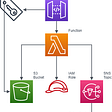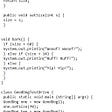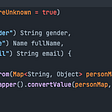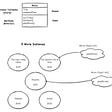# A walk Through Math Class in Java

The Math class is present in java.lang package, this is a final class and all the methods present in Math class are static so we need not create an object to Math class to call them,

public final class Math extends Object{}

The Math class contains methods for performing basic numeric operations such as the elementary exponential, logarithm, square root, and trigonometric functions.

Here is the list of general purpose methods which are present in Math class

static double sin(double d) → This method returns the sin value of the d here d is in radians

static double cos(double d) → This method returns the cosine value of the d here d is in radians

static double tan(double d) → This method returns the tangent value of the d here d is in radians

static double log(double d) → This method returns the natural logarithmic value(base e) of d

static double log10(double d) → This method returns the base 10 logarithmic value of the d

static double pow(double d1,double d2) → This method returns the value of d1 to the power d2

static double sqrt(double d) → This method returns the square root value of the d

static double abs(double d) → This method returns the absolute value of the d

static double ceil(double d) → This method raises the given value to next integer value and if the integer value is passed to it then it returns the same integer value

static double floor(double d) → This method decreases the given value to previous integer value and if the integer value is passed to it then it returns the same integer value

static double round(d) → It returns the rounded value of d, if the fraction part is more or equal to 0.5 then 1 is added to integer part otherwise same integer part is returned

static double random() → This method returns a random number between 0 to 1

static double max(d1,d2) → It returns the max value of d1 and d2

static double min(d1,d2) → It returns the min value of d1 and d2

Lets test all these methods in a program:

package com.exercise;

public class MathClass {

public static void main(String[] args) {

double sin = Math.sin(0.5);

System.out.println(sin); // 0.479425538604203

double cos = Math.cos(0.5);

System.out.println(cos);// 0.8775825618903728

double tan = Math.tan(0.5);

System.out.println(tan); // 0.5463024898437905

double log = Math.log(2);

System.out.println(log); // 0.6931471805599453

double log10 = Math.log10(2);

System.out.println(log10); // 0.3010299956639812

double pow = Math.pow(2, 3);

System.out.println(pow); // 8.0

double sqrt = Math.sqrt(25);

System.out.println(sqrt); // 5.0

double exp = Math.exp(2);

System.out.println(exp); // 7.38905609893065

double abs = Math.abs(-189.23);

System.out.println(abs); // 189.23

double ceil = Math.ceil(4.5);

System.out.println(ceil); // 5.0

double floor = Math.floor(4.5);

System.out.println(floor); /// 4.0

long round = Math.round(145.567);

System.out.println(round); // 146

double random = Math.random();

System.out.println(random); // 0.5178217441160299

int max = Math.max(100, 200);

System.out.println(max); // 200

int min = Math.min(100, 200);

System.out.println(min); // 100

}

}

Thats all for this Math class.. See you in the next post.. Keep learning…

--

--

--

## More from Abhishek Singh

Engineer | Blogger | Traveller

Love podcasts or audiobooks? Learn on the go with our new app.

## HeroesTD GIVEAWAY — 3 LUCKY SLOTS TO BE WHITELISTED TO BUY AN NFT BOX## My Journey to Web Development Bootcamp.## Role of PSD to HTML Conversion in the Success of a Website## Transfer Files to S3 Using REST API## Deploying multi tier VPC through Terraform.## 9 Concepts for Every Python Programmer## Software Design Patterns for Human Relationships## Developing and Deploying with Docker## Abhishek Singh

Engineer | Blogger | Traveller

## BEHAVIOUR OF THE OBJECT## JVM and JDK## Java record: A Brief## Digging into JAVA — Chapter 04